# Test:Triangles- 1

## 25 Questions MCQ Test Mathematics (Maths) Class 9 | Test:Triangles- 1

Description
This mock test of Test:Triangles- 1 for Class 9 helps you for every Class 9 entrance exam. This contains 25 Multiple Choice Questions for Class 9 Test:Triangles- 1 (mcq) to study with solutions a complete question bank. The solved questions answers in this Test:Triangles- 1 quiz give you a good mix of easy questions and tough questions. Class 9 students definitely take this Test:Triangles- 1 exercise for a better result in the exam. You can find other Test:Triangles- 1 extra questions, long questions & short questions for Class 9 on EduRev as well by searching above.
QUESTION: 1

Solution:
QUESTION: 2

Solution:
QUESTION: 3

### In the adjoining figure, the rule by which △ABC ≅ △ADC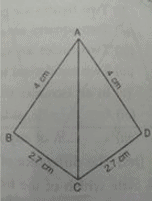Solution:
QUESTION: 4

In the adjoining figure, BC = AC. If ∠ACD = 115, the ∠A is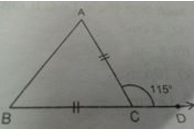Solution:
QUESTION: 5

If the bisector of the angle A of a △ABC is perpendicular to the base BC of the triangle then the triangle ABC is :

Solution:
QUESTION: 6

Which is true?

Solution:
QUESTION: 7

If ABC and PQR are similar triangles in which ∠ A = 470 and ∠ Q = 830, then ∠ C is: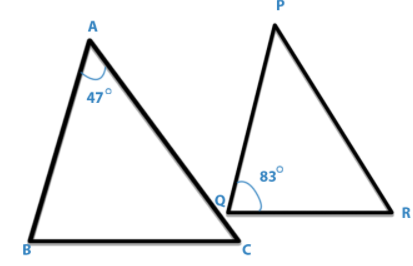Solution:
QUESTION: 8

In the adjoining figure, BC = AD, CA⊥AB and BD⊥AB. The rule by which △ABC ≅ △BAD is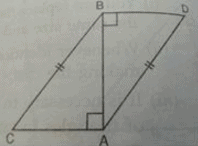Solution:
QUESTION: 9

In the given figure, ABC is an equilateral triangle. The value of x+y is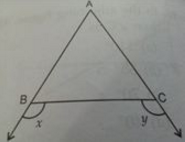Solution:
QUESTION: 10

In quadrilateral ABCD, BM and DN are drawn perpendiculars to AC such that BM = DN. If BR = 8 cm. then BD is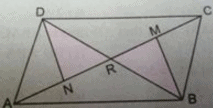Solution:
QUESTION: 11

If the altitudes from vertices of a triangle to the opposite sides are equal, then the triangles is

Solution:
QUESTION: 12

In △AOC and △XYZ, ∠A =∠X, AO = YZ, AC = XY, then by which congruence rule △AOC ≅ △XYZ

Solution:
QUESTION: 13

In the adjoining figure, AB = AC and AD⊥BC. The rule by which △ABD ≅ △ACD is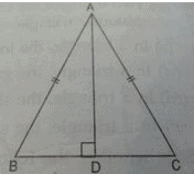Solution:
QUESTION: 14

In the adjoining Figure, AB = AC and BD = CD. The ratio ∠ABD : ∠ACD is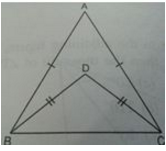Solution:
QUESTION: 15

In △ABC and △PQR, three equality relations between corresponding parts are as follows: AB = QP, ∠B = ∠P BC = PR. State which of the congruence criterion applies in this case:

Solution:
QUESTION: 16

O is any point in the interior of △ABC.Then which of the following is true?

Solution:
QUESTION: 17

It is not possible to construct a triangle when its sides are:

Solution:
QUESTION: 18

In the adjoining figure, AB = AC and AD is bisector of ∠A. The rule by which △ABD ≅ △ACD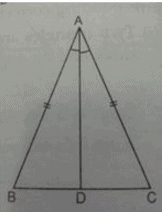Solution:
QUESTION: 19

In the adjoining figure, AB⊥BE and FE⊥BE. If AB = FE and BC = DE ,then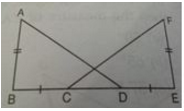Solution:
QUESTION: 20

D,E and f are the mid-points of the sides BC, CA and AB res. Of △ABC. Then △DEF is congruent to triangle:

Solution:
QUESTION: 21

Which of the following is not a criterion for congruence of triangles?

Solution:
QUESTION: 22

△ABC = △PQR, then which of the following is true?

Solution:
QUESTION: 23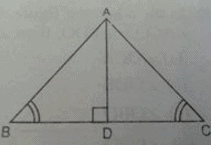Solution:
QUESTION: 24

In the adjoining figure, ABCD is a quadrilateral in which BN and DM are drawn perpendiculars to AC such that BN = DM. If OB = 4 cm. then BD is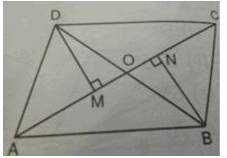Solution:
QUESTION: 25

In triangle PQR length of the side QR is less than twice the length of the side PQ by 2 cm. Length of the side PR exceeds the length of the side PQ by 10 cm. The perimeter is 40 cm. The length of the smallest side of the triangle PQR is :

Solution: